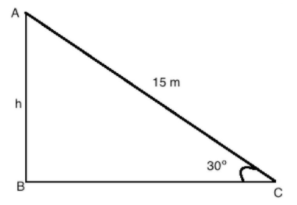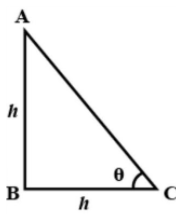QuestionAnswers

# Statement 1- A kite is flying with the string inclined at $30^\circ$ to the horizontal. The height of the kite above the ground when the string is 15 meter long is 7.5 meters.Statement 2- The altitude of the sun is $45^\circ$, when the length of the shadow of a pole is the same as the height of the pole.Which of the above statements is/are true?A. Only 1B. Only 2C. Both 1 and 2D. Neither 1 nor 2

Hint: To solve this, we need to check the statements. In statement 1, inclined length and string lengths are given from which we can derive height.
In statement 2, angle of elevation is asked and base and height are equal. From that we can derive an angle of elevation.

Let’s check if statement 1 is true or false.
Representing the data of statement 1 in below diagram,Data given in the statement 1,
A kite is flying with the string inclined at $30^\circ$ to the horizon i.e.
$\sin A = 30^\circ$
String length AC = 15 meter
We have to check the height of the kite above the ground is 7.5 meter
i.e. AB = 7.5 meter
So, we have $\sin A = 30^\circ$
Hence,
$\Rightarrow \dfrac{h}{{15}} = \sin 30^\circ$
Putting value of $\sin 30^\circ$ in it,
$\dfrac{h}{{15}} = \dfrac{1}{2}$
$\Rightarrow h = \dfrac{{15}}{2} = 7.5$ meters
Hence the height of kite above the ground is 7.5 meters
And statement 1 is true.
Now, let’s check if statement 2 is true or false.
Representing the statement 2 in below diagram,Let height of the pole be AB = h
According to the statement 2 height of the pole is equal to the shadow of the pole BC
Then BC = h
Let $\theta$ be the angle of elevation
According to the diagram,
$\tan \theta = \dfrac{{AB}}{{BC}}$
Putting the value of AB and BC in it we get,
$\tan \theta = \dfrac{h}{h} = 1$
Or, $\theta = {\tan ^{ - 1}}1 = 45^\circ$
Hence the angle of elevation is $45^\circ$ that means the altitude of the sun is $45^\circ$.
The statement 2 is also true.
Both statements are true.

So, the correct answer is “Option C”.

Note: The angle of elevation is the altitude of the sun.
You might make mistakes while making inverse trigonometry.
Both the statements are represented in a right angled triangle.